MEDIUM

# Find Leaves of a Binary Tree: Problem + Solution in Java and Python

Written ByTom WagnerClumsy Penguin

## Introduction to the Find Leaves of Binary Tree Problem

The Find Leaves of Binary Tree problem involves sequentially identifying all of the leaf nodes in a binary tree and returning their values. As with many tree problems, the solution requires the application of a traversal algorithm that visits nodes in the order most appropriate for this task, allowing for a linear time complexity.

## Example of the Find Leaves of a Binary Tree Interview Question

Given a binary tree, extract all the leaves in repeated succession into a list of lists by starting at the bottom and working your way upwards.

Input: Given the following TreeNode structure:

``````# Definition for a binary tree node.
# class TreeNode:
#     def __init__(self, val=0, left=None, right=None):
#         self.val = val
#         self.left = left
#         self.right = right``````

And given the following tree: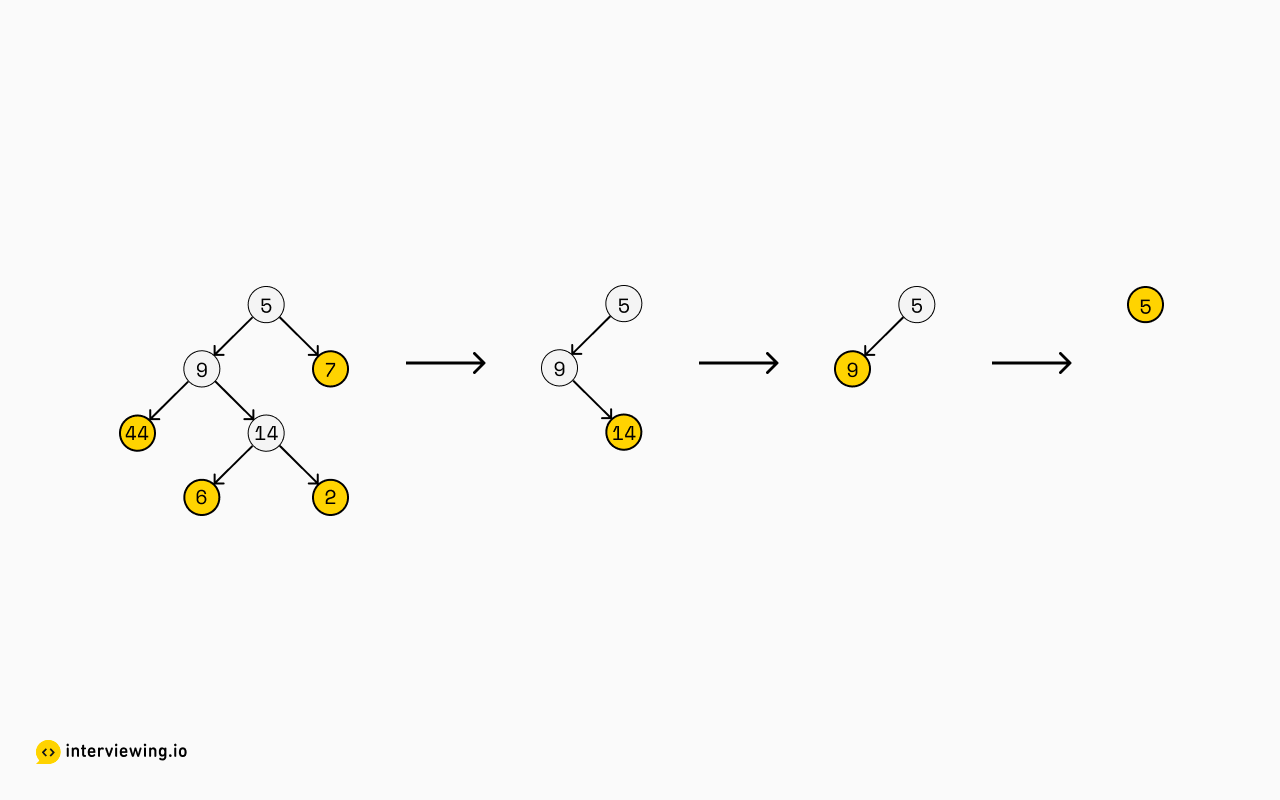``````[
[44, 6, 2, 7],
,
,

]``````

## 2 Ways to Find Leaves of a Binary Tree

The first things that ought to come to mind when thinking about trees are the approaches in which we can use to traverse them. There are pre-, in-, and post-order traversals, and also depth-first and breadth-first approaches. Perhaps asking the interviewer a little more might help us consider which of these will get us closer to the answer, and which will not.

Does the order of the elements in the sub-lists matter? Is it alright to return the same result but with shuffled elements?

Ideally please keep it "left-to-right" as shown in the example, but if that's a blocker for you right now, go ahead and solve it with the result in any order and we can revisit this point.

Perhaps the interviewer will be lax about this at the outset, in the desire to get something working first and improving on it later.

### Approach 1: Level-by-level

At this point, we can stare at the tree and think about various ways to do a traversal and what that might result in. The first thing that might come to mind, is that it looks a lot like a level-by-level extraction: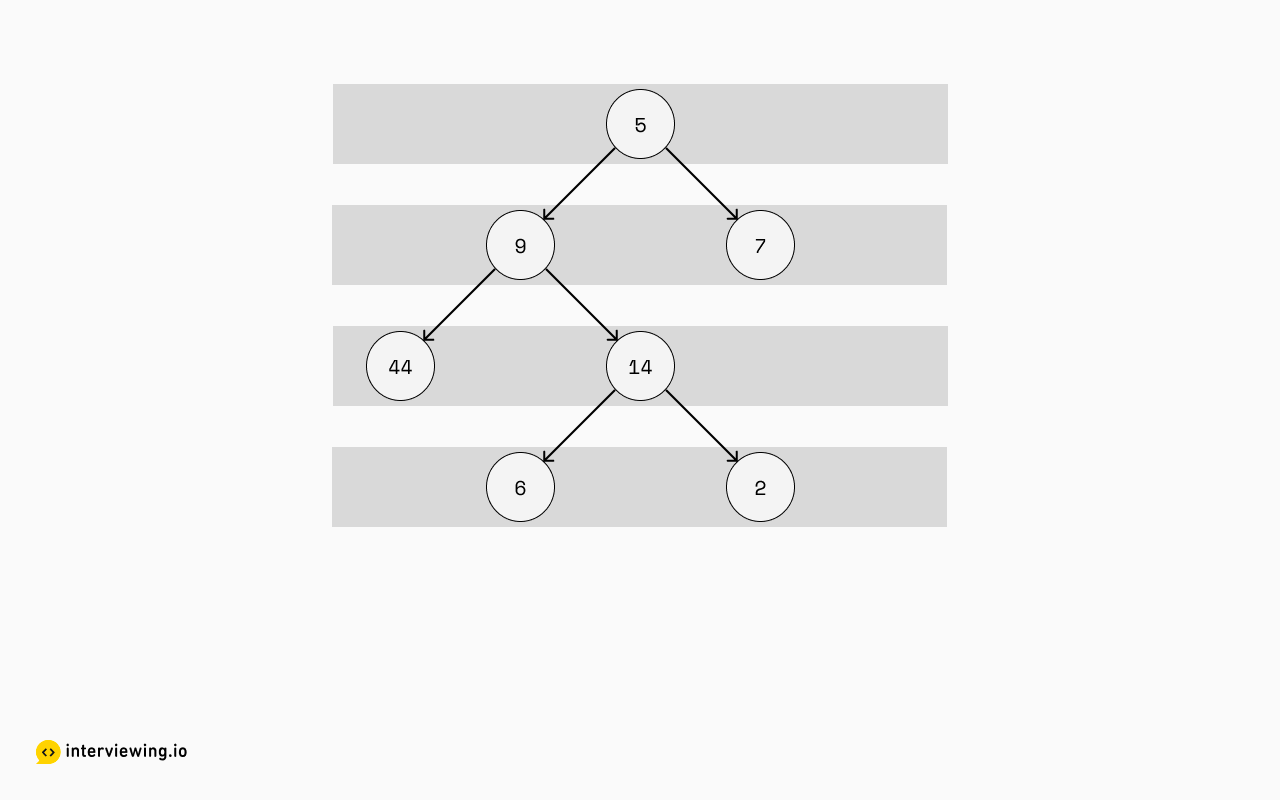But that isn't exactly right. That'd give us this output:

``````[
,
[9, 7],
[44, 14],
[6,2],
]
``````

If you reverse the order of elements here, it gets rather close:

``````[
[6,2],
[44, 14],
[9, 7],

]
``````

But it still isn't there yet. Let's look closer at the first sub-list -- what we want is to group the elements more like this: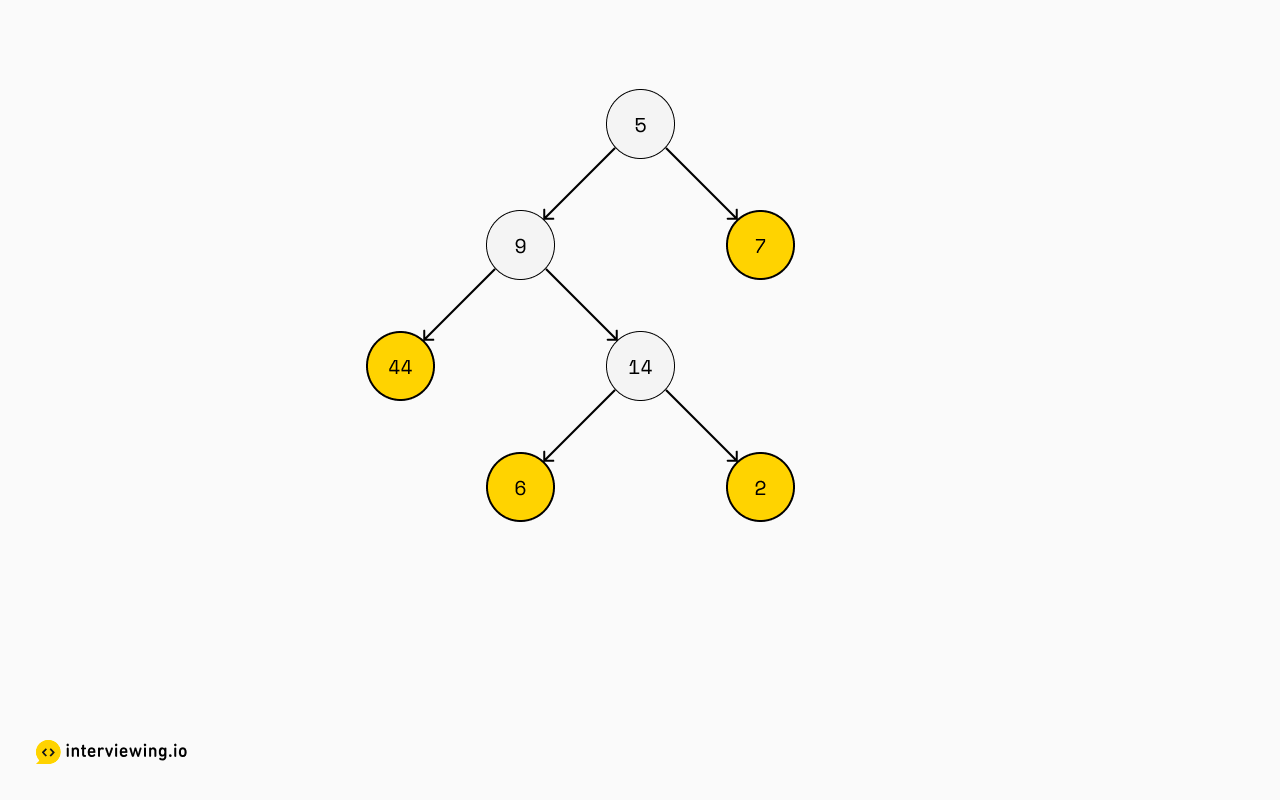This becomes the first sub-list in our result, and it's extracted by grabbing all elements, left-to-right, that don't have any children. We'll have this result so far:

``````[
[44, 6, 2, 7]
]
``````

Let's take a look at the next sub-list: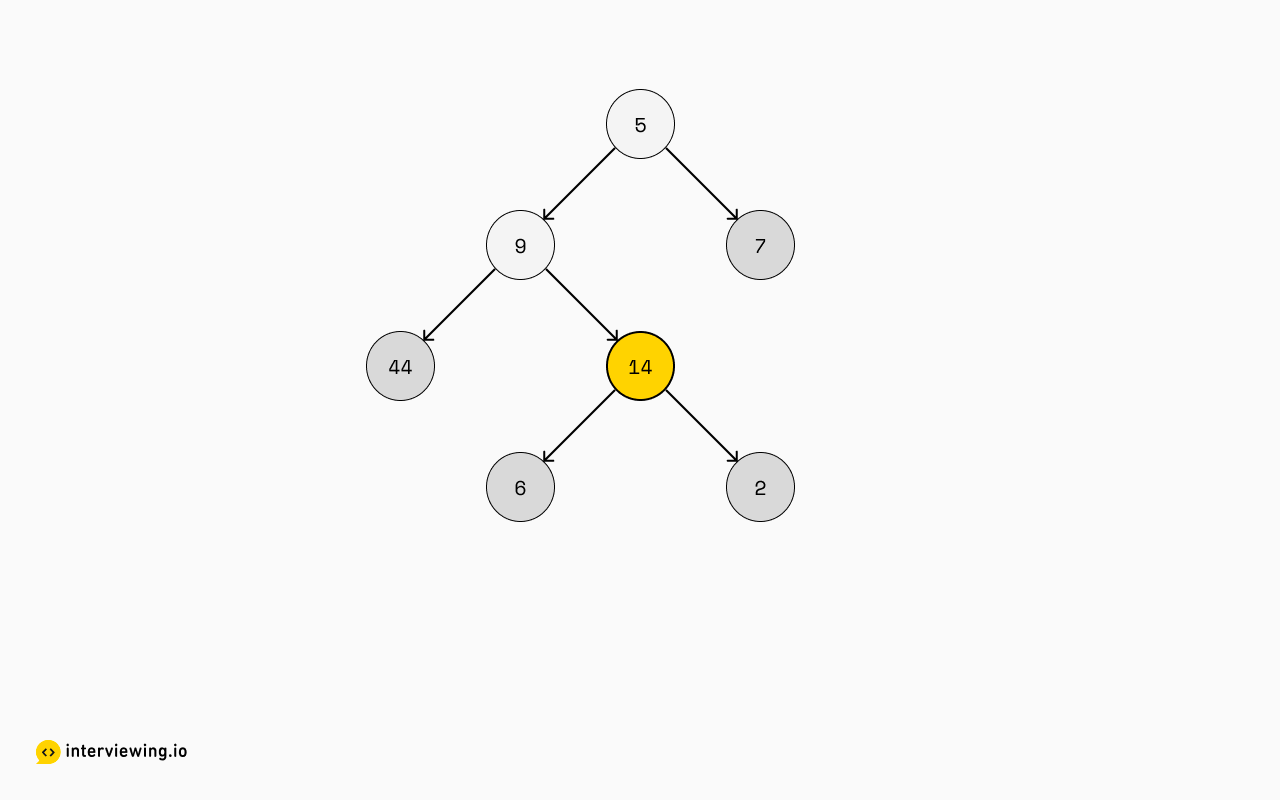We'll now give our attention to `14` but not `5` or `9`. We can suspect that they're being excluded in this step because not all of their children have been processed yet, and `14` is being processed because all of their children have been processed.

``````[
[44, 6, 2, 7],
,
]
``````

One more step here, we'll turn to `9` now that `14` has been dealt with. We still haven't reached `5` yet: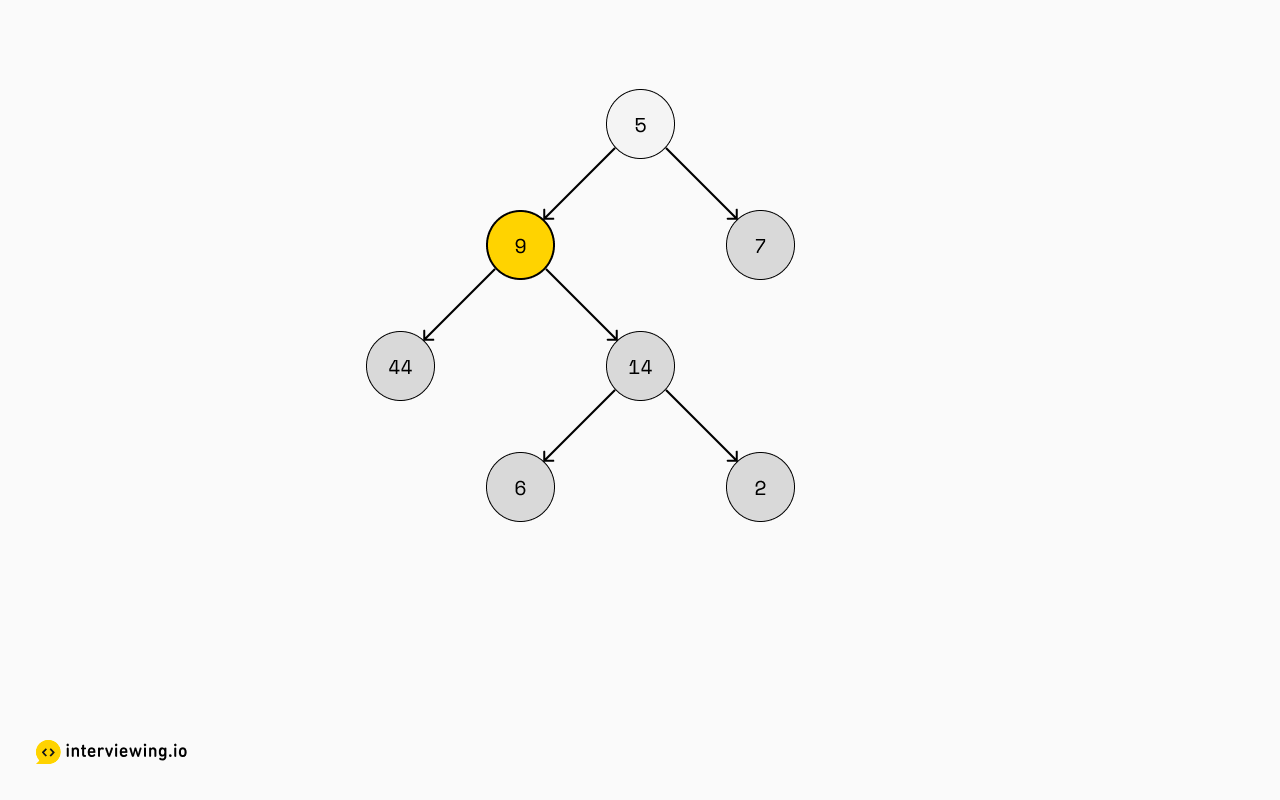``````[
[44, 6, 2, 7],
,

]
``````

Lastly, we'll work on the root, `5`: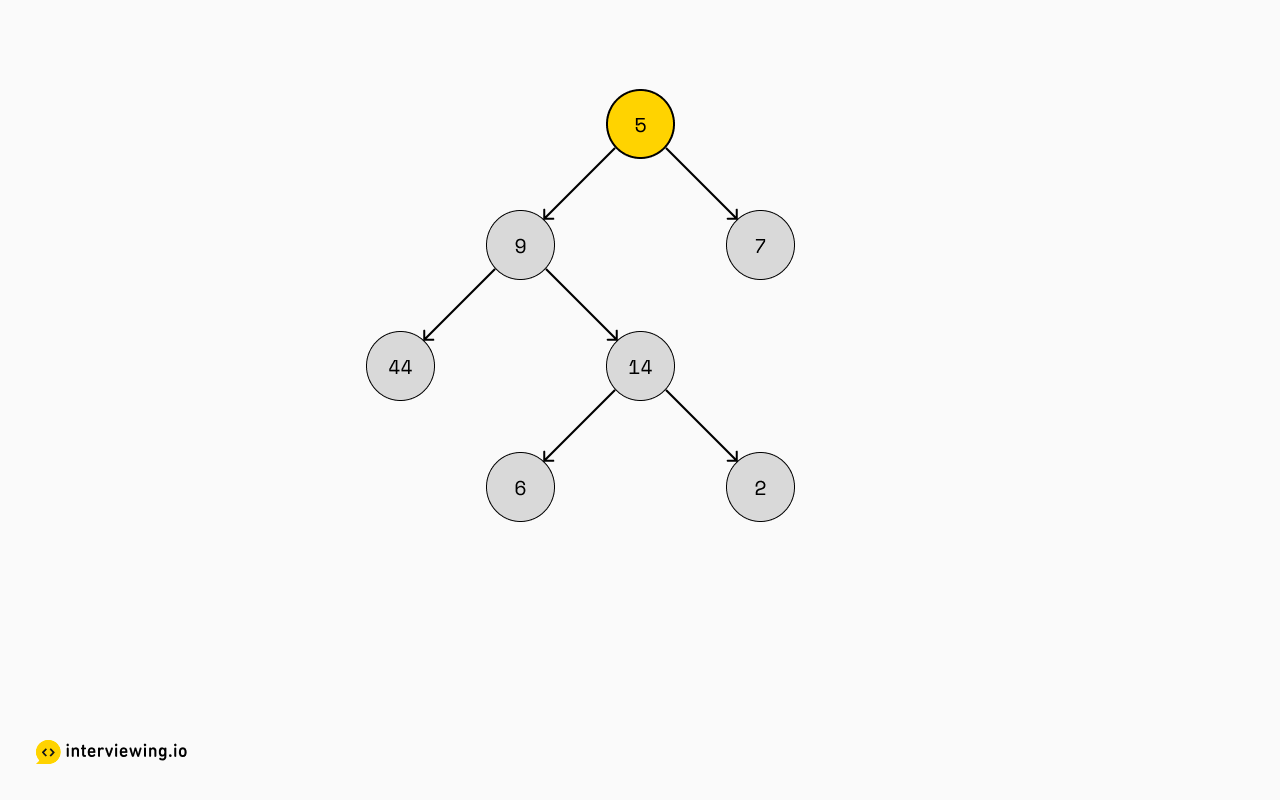Giving us our final result:

``````[
[44, 6, 2, 7],
,
,

]
``````

### Approach 2: Depth-First Search (DFS)

So now that we’ve identified the need to focus on child nodes first we need to select an approach that will accomplish this, and the approach best-suited for this task is called depth-first search (DFS).

Further, there are multiple types of DFS algorithms: pre-order, in-order and post-order. “Pre-”, “in-” and “post-” here refer to when a node should be processed relative to its children: “Pre-order DFS” means that a node should be processed prior to traversing to its children “In-order DFS” means that a node should be processed after processing its left child but before processing its right child “Post-order DFS” means that a node should be processed after processing both of its children (and therefore any of its grandchildren, great-grandchildren, etc.)

Given we want to process all nodes without children first, it should be clear which flavor of depth-first search is the right approach here: post-order.

If we consider the indexes that each element ends up in, we can somewhat see that it's almost like constructing the height of a tree, but from the bottom-up: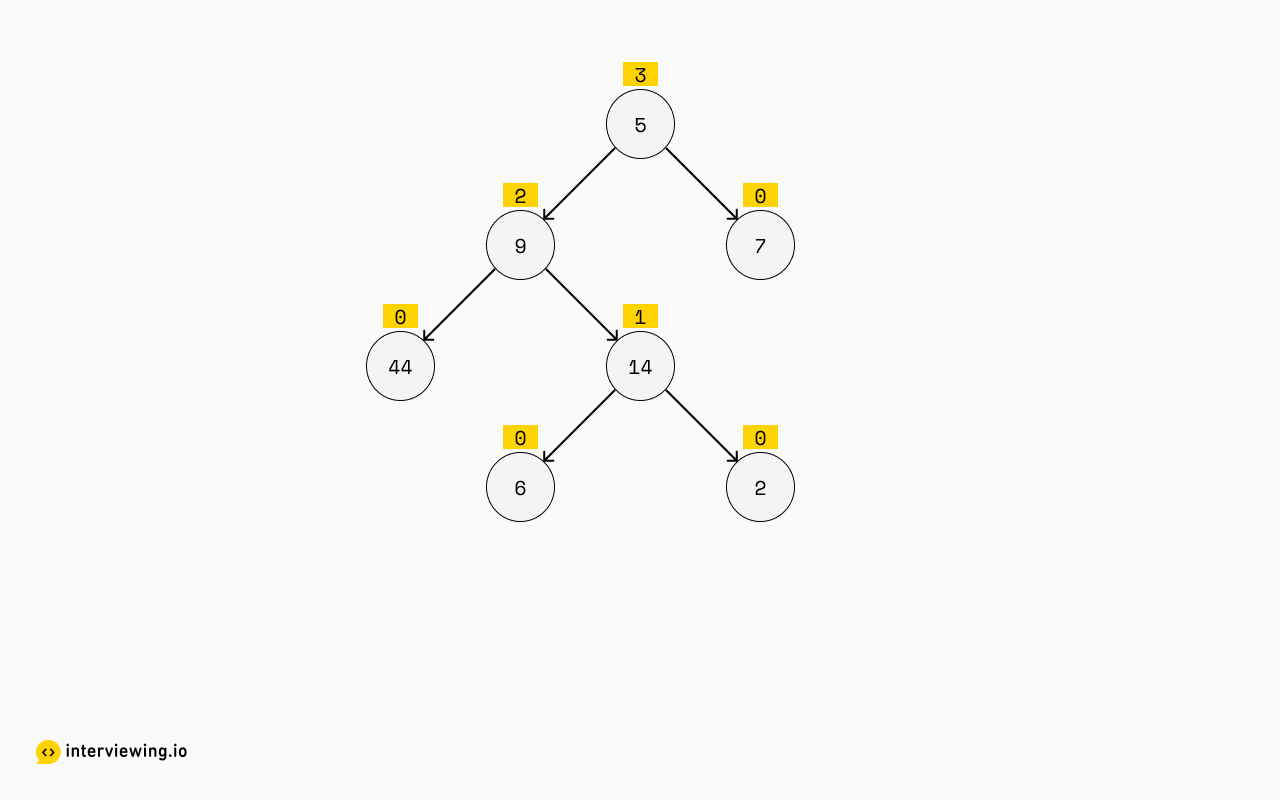It seems that:

• Nodes with no children and proper leaves will always have a height of zero and a sublist index of zero.
• The height of all other nodes will be derived from the heights passed upwards from their children. If their children have different heights, the greater of the two heights "wins" and the parent node’s height will equal the winner's height plus one. That will also be the sublist index the node value ends up in.

This is enough to begin building the solution. We'll write a depth-first traversal, visiting the left child, then the right child, and have children report their heights up to their parents.

#### Extract Leaves Python Solution - Depth-first Search

``````#!/usr/env python3
from dataclasses import dataclass
from typing import List

@dataclass
class Node:
val: int = 0
left: 'Node' = None
right: 'Node' = None

# our solution
def extractLeaves(root: Node):

def dfs(n: Node):
# Handle leaf and edge cases nicely, so we can call `max` unconditionally
if not n:
return -1

# Our height is the maximum of our children's height - plus one for ourself.
h = max(dfs(n.left), dfs(n.right)) + 1

# Ensure that our answer list is long enough for us to insert

# Insert ourselves into the correct sublist

# report our height up to our parent
return h

# run the dfs over our tree
dfs(root)

def test(input: Node, desired: List[List[int]]):
out = extractLeaves(input)
if out == desired:
print(f"PASS: {input} -> {desired}")
else:
print(f"FAIL! {input} -> {out}, expected {desired}")

def runTests():
testTree = Node(5, Node(9, Node(44), Node(14, Node(6), Node(2))), Node(7))

test(None, [])
test(Node(5), [])
test(testTree, [[44, 6, 2, 7], , , ])

runTests()
``````

#### Time/Space Complexity

• Time Complexity: `O(n)`. Our algorithm visits each node exactly once, and does a constant amount of work per node. We can say that our time complexity is `O(n)`, where `n` is the number of nodes in the tree.
• Space Complexity: `O(n)`. Our algorithm makes a copy of all of the values of the tree as it assembles its answer, which is `O(n)`. It also does a depth-first search to process the input, which takes `O(h)` space, where `h` is the height of the tree. If the tree is a balanced binary tree, then `h` is the base-2 logarithm of `n`, or `lg(n)`. If the tree is not a balanced binary tree the worst-case space complexity `O(h)` == `O(n)`, as the height of a tree with all nodes on one side could equal the size of the tree as a whole.

## Practice the Find Leaves of a Binary Tree Problem With Our AI Interviewer

Start AI Interview